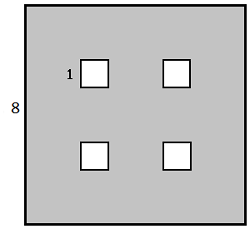In the figure above, the four small squares are congruent. What is the area of the shaded region?

(A) $\ \ 16$
(B) $\ \ 48$
(C) $\ \ 60$
(D) $\ \ 63$
(E) $\ \ 68$

×